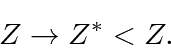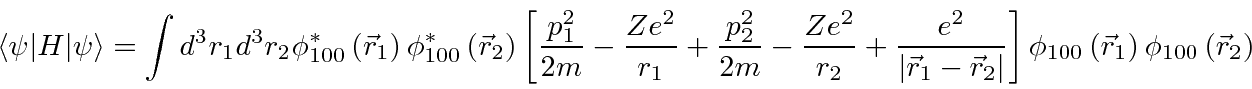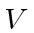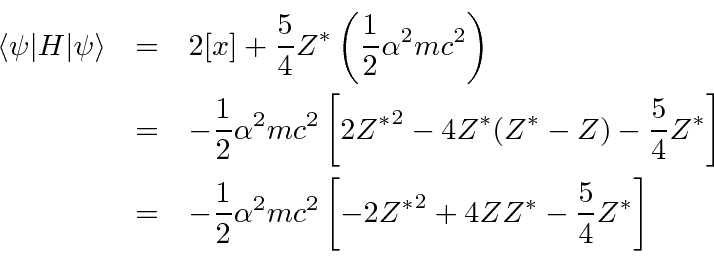## Variational Helium Ground State Energy

We will now add one parameter to the hydrogenic ground state wave function and optimize that parameter to minimize the energy. We could add more parameters but let's keep it simple. We will start with the hydrogen wavefunctions but allow for the fact that one electron screens'' the nuclear charge from the other. We will assume that the wave function changes simply by the replacementOf course thein the Hamiltonian doesn't change.

So our ground state trial function isMinimize the energy.We can recycle our previous work to do these integrals. First, replace theinwith aand put in a correction term. This makes thepart just a hydrogen energy. The correction term is just a constant overso we can also write that in terms of the hydrogen ground state energy.Then we reuse the perturbation theory calculation to get theterm.Use the variational principle to determine the best.Putting these together we get our estimate of the ground state energy.Now we are within a few percent. We could use more parameters for better results.

Jim Branson 2013-04-22